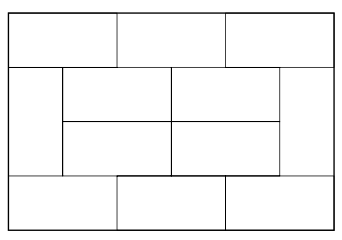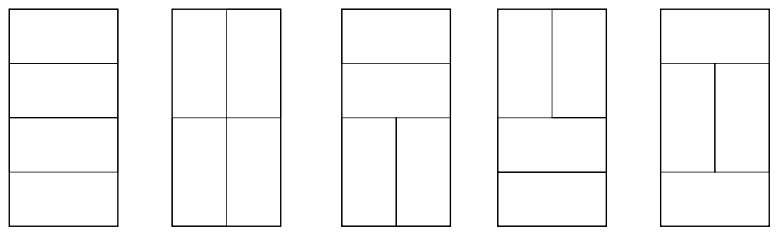시간 제한메모리 제한제출정답맞힌 사람정답 비율
1 초 128 MB218844.444%

## 문제

You are given a set of 2n dominos, where n < 1000 is an integer. The dominos have width 1 and length 2. It is possible to arrange these dominos in such a way that they form a 4 × n rectangle. For instance, in Figure 1, we have arranged 12 dominos to form a 4 × 6 rectangle.Figure 1: Example with n = 6.

In fact, where n > 1, there are several ways of arranging dominos to form a 4 × n rectangle. For instance, in Figure 2, you can see the 5 different ways of forming a 4 × 2 rectangle:Figure 2: The 5 different ways of forming a 4 × 2 rectangle.

We denote by Rn the number of different ways of forming a 4 × n rectangle with 2n dominos. For instance, R2 = 5, as you can see in Figure 2. As Rn can be quite large, even for small values of n, you are only asked to compute the last three digits of Rn. Your program should output the value of the last three digits of Rn without leading zeros. For instance, R17 = 26915305, so when n = 17, your program should output 305 (the last three digits). Another example, R2 = 5 (or 005), so when n = 2, your program should output 5. Should the last three digits be zeros for some Rn, your program should simply output 0.

## 입력

The input contains the integer n. Remember that n < 1000.

## 출력

You should write an integer giving the value of the last three digits of Rn without leading zeros in the output. (Note that the output value is simply the value Rn mod 1000; that is, the remainder of Rn divided by 1000.)

## 예제 입력 1

2


## 예제 출력 1

5


## 예제 입력 2

17


## 예제 출력 2

305